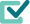# Your Digital Authoring and Assessment Tool built to empower STEM education

Welcome to the WirisQuizzes demo, experience a new way of building online assessments and teaching science and math. Play around with our demo exercises — from the most simple to the most advanced.
Handwritten input recognition
Powerful Math Engine (CAS)
Customize Feedback

## Open Questions

Our mathematical engine automatically compares the correct answer to the student's answer and grades it accordingly
Isolate the variable $y$ in $y-x=2$: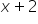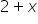Student answer is equivalent to correct answer
Calculate the sum $\frac{1}{4}+\frac{1}{4}$:Student answer is mathematically equal to correct answer

## Evaluation Rules

Instructors can select rules for evaluation and our tool automatically validates these additional grading properties
Simplify the fraction $\frac{2}{4}$: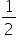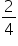Simplification Rules
Calculate the sum $30\mathrm{cm}+500\mathrm{mm}$: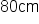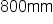Unit Rules

## Dynamic Questions with Random values

We help prevent cheating by allowing you to use random parameters in your assessment questions and create dynamic questions
Add two random fractions: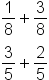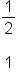Random Parameters Input
Interpret this bar chart: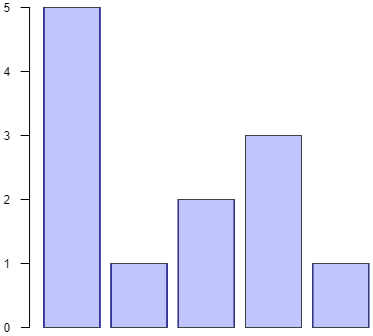Random Parameters Input
Statistics

Students can answer questions with an interactive graphical representation
Draw the bisector of the highlighted angle.
Student's Toolbar Customization
Fill the region delimited by
Highlight areas of the graph
Allows for degree of tolerance for accepted graphical errors

Our engine understands all levels of maths: from K-12 all the way to college
Statement
Give a basis of the (randomly computed) subspace   $F=\left\{\left(x,y,z\right)\in {\mathrm{ℝ}}^{3}: 2x+y+z=0\right\}$
$\left\{\left[1,-1,-1\right],\left[1,0,-2\right]\right\}$
$\left\{\left[1,-1,-1\right],\left[1,-2,0\right]\right\}$
$\left\{\left[1,0,-2\right],\left[1,-2,0\right]\right\}$
$\left\{\left[3,-2,-4\right],\left[-2,5,-1\right]\right\}$
... and any mathematically correct answer!
Random Parameters Input
Multi-part questions
Statement
Give a solution for the following ODE:   $y\text{'}+\mathrm{sin}\left(x\right)y={\mathrm{e}}^{\mathrm{cos}\left(x\right)}$
$x{\mathrm{e}}^{\mathrm{cos}\left(\mathrm{x}\right)}$
$\left(x+1\right){\mathrm{e}}^{\mathrm{cos}\left(\mathrm{x}\right)}$
$\left(x+k\right){\mathrm{e}}^{\mathrm{cos}\left(\mathrm{x}\right)}$
... and any mathematically correct answer!

## Fill in the Blank Questions

Create fill in the blank STEM questions with multiple gaps
Given the following function, fill in the blanks in the table: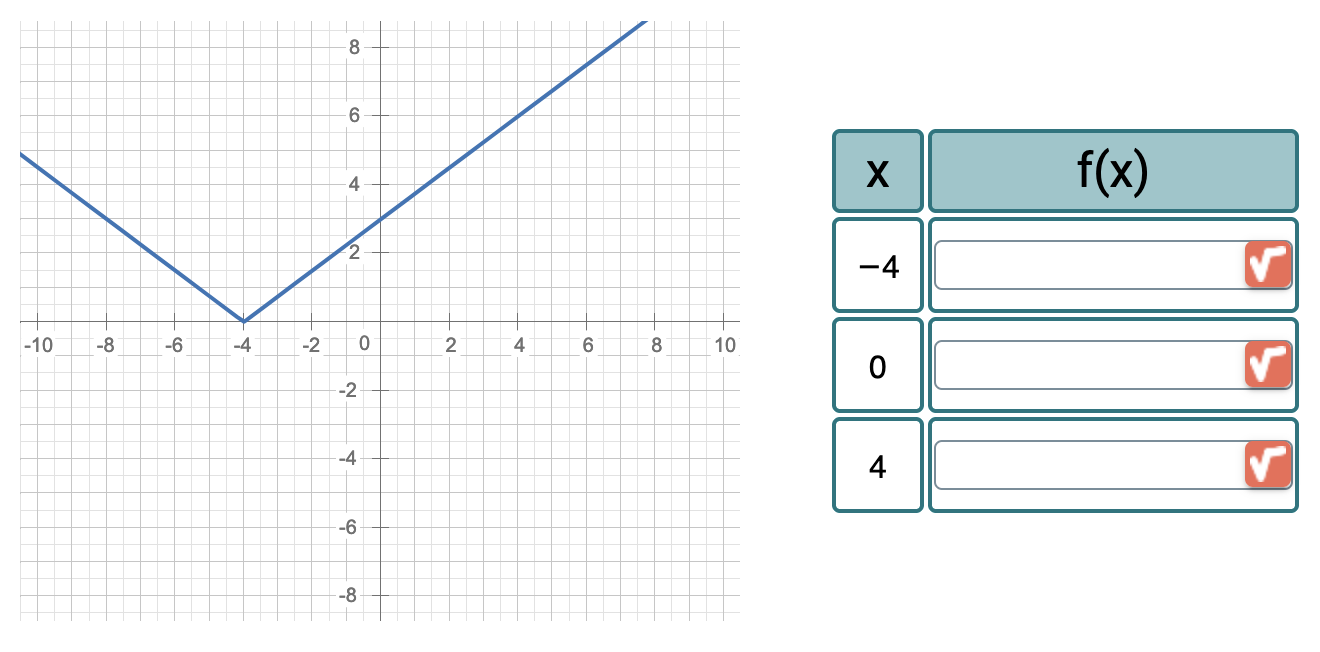Graphics in the question text

## Powered byYour auxiliary digital STEM whiteboard and calculator

## Statistical Questions new

Our graphic tool also allows for representing statistical charts that are interactive for the student
Group the number of rainy days over the year 2020 in Barcelona into groups of three months, according to the following data:
 Jan Feb Mar Apr May Jun Jul Aug Sep Oct Nov Dec 5 6 9 16 12 17 11 13 16 17 13 10
Interactive Statistical Charts
Bar Chart
After the elections for the delegate of a class, Alice recieved 13 votes, Bob 9 and Charlie 4.

Represent the results of the election using the following pie chart:
Interactive Statistical Charts
Pie Chart

# Available in Your LMS